# 1D and 2D Gaussian Derivatives

http://campar.in.tum.de/Chair/HaukeHeibelGaussianDerivatives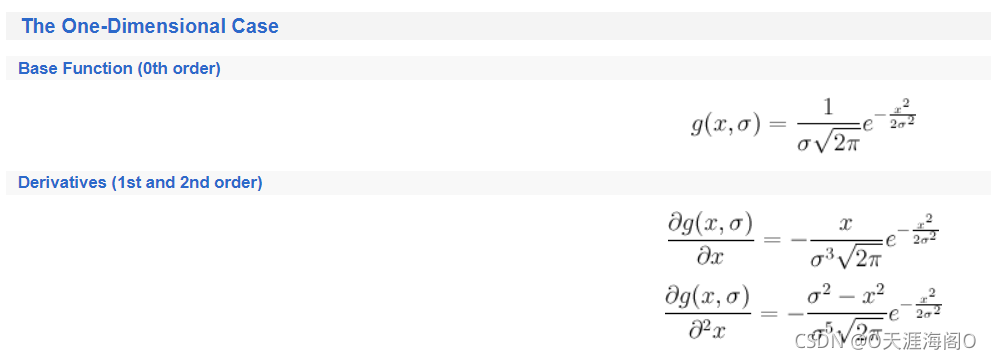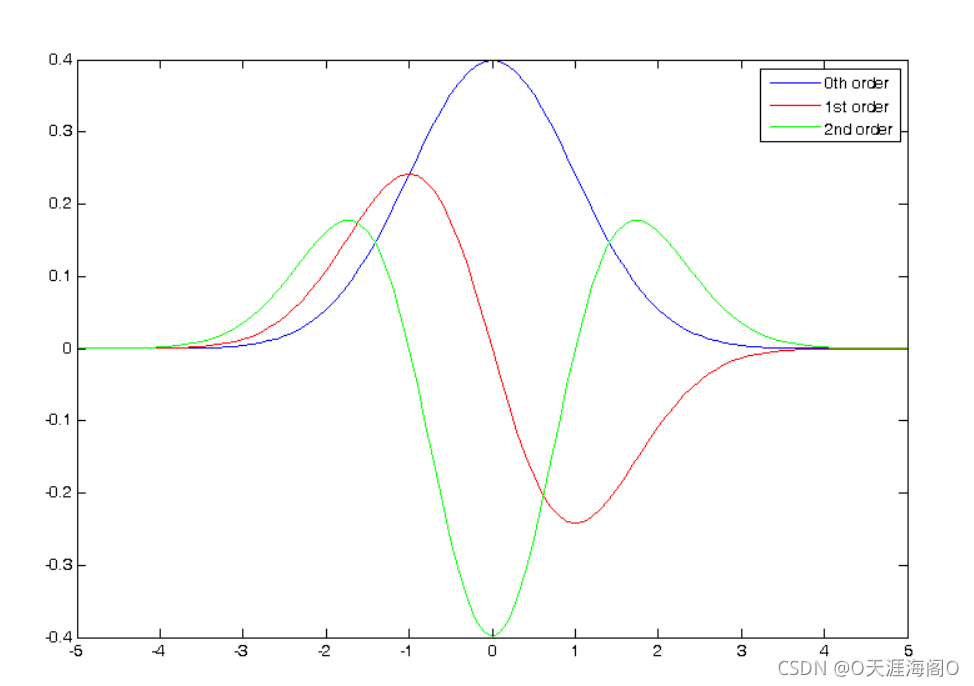The Two-Dimensional Case
Base Function (0th order)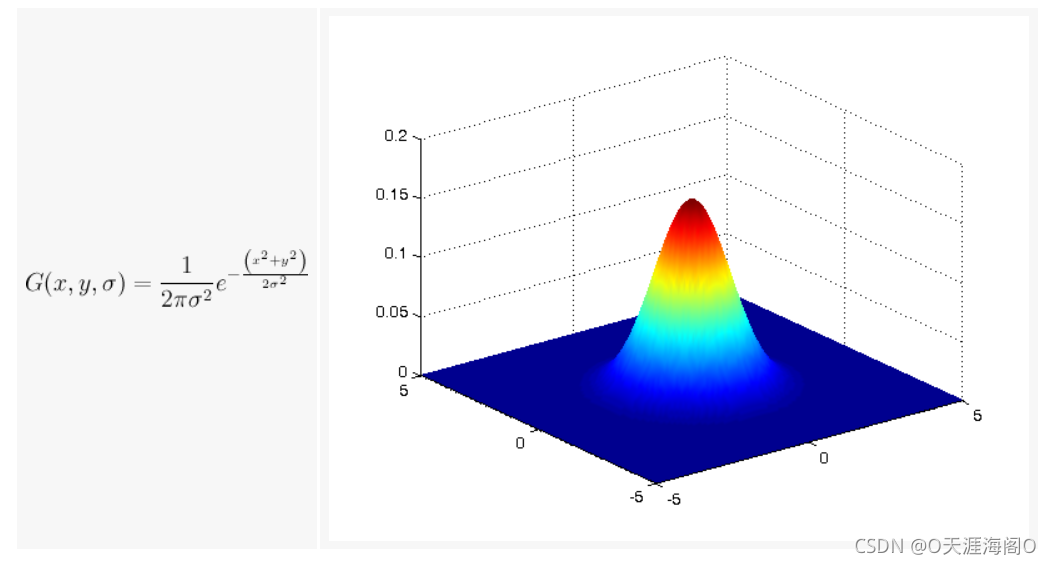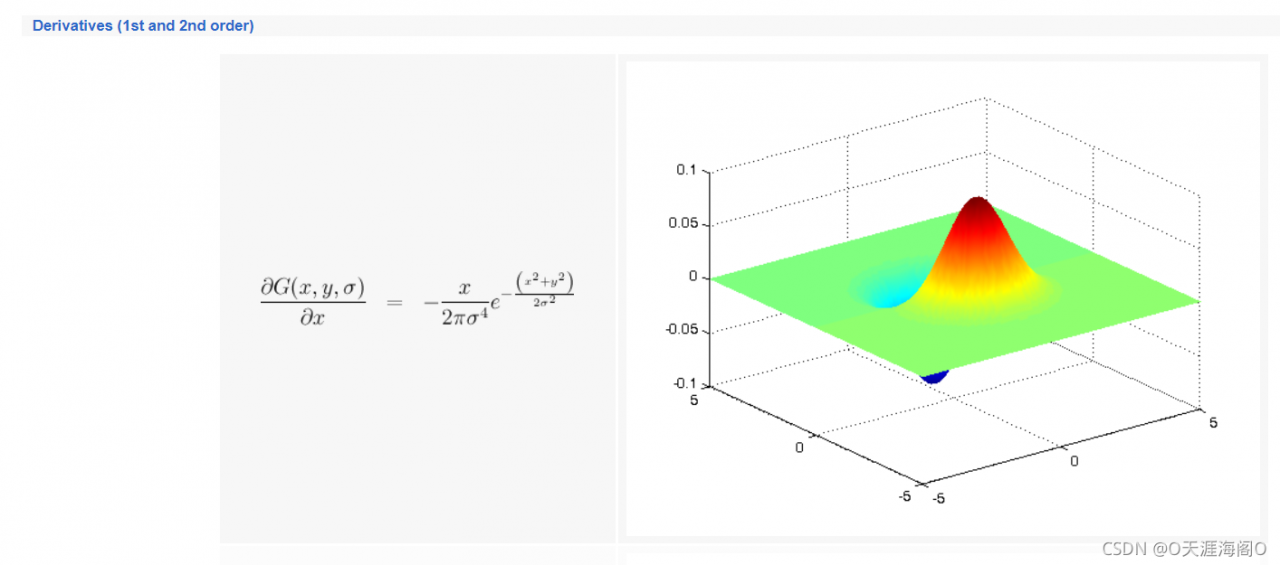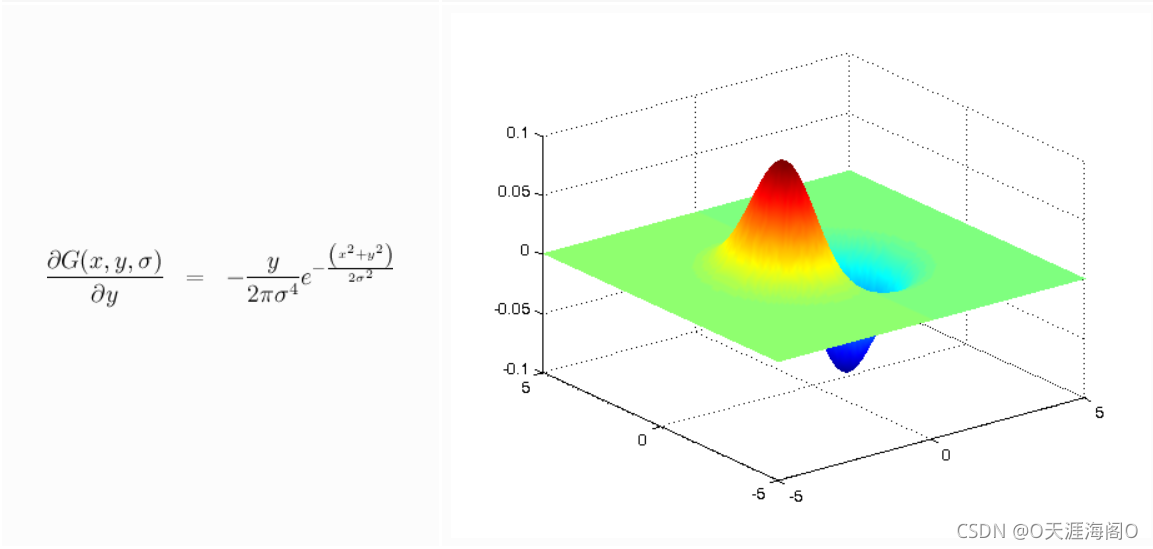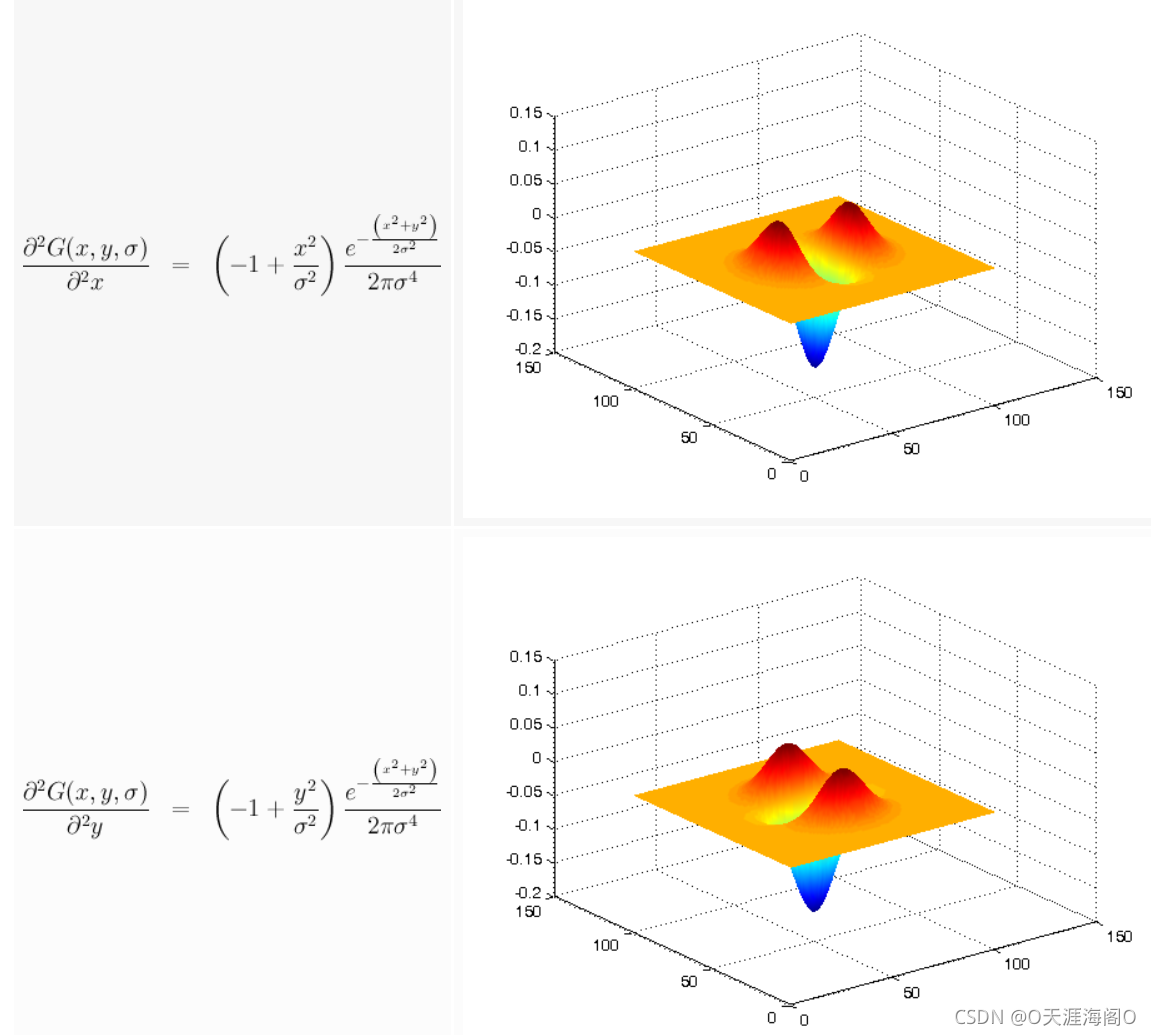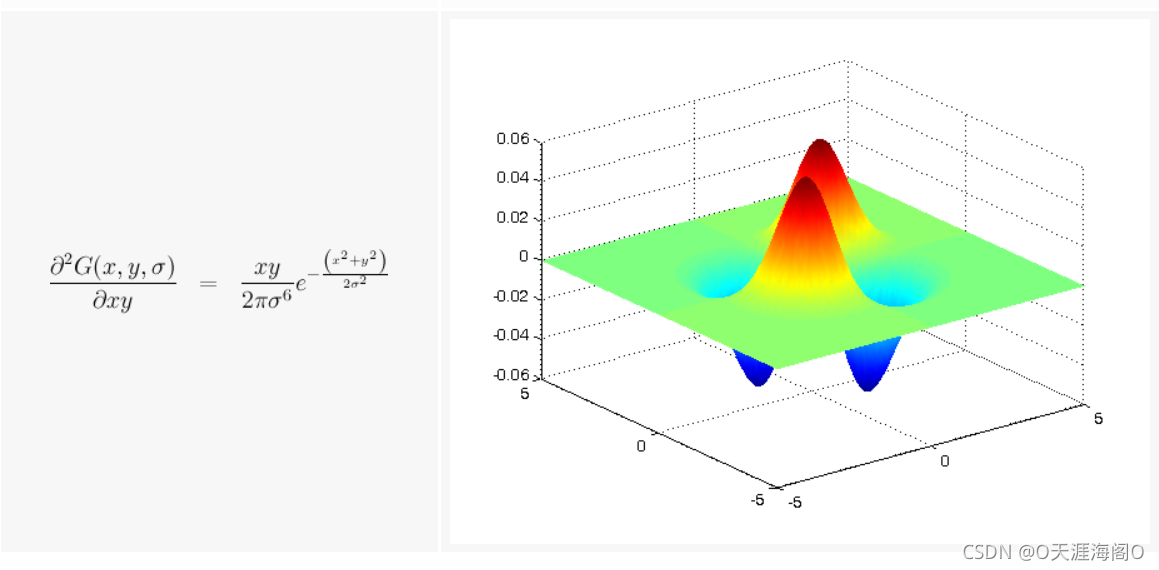Computes discrete 1D gaussian functions

``````function [ gaussian ] = gaussian( x, sigma, order, normalize )
if isempty(normalize)
normalize = false;
end

gaussian_base = exp(-(x.*x)/(2*sigma^2));

% we will do the same as ITK. this is just done to be able to
% compare gaussian results with those coming from ITK.
if (normalize)
gaussian_base = sigma*gaussian_base;
end

if order == 0
gaussian = 1/(sigma*sqrt(2*pi)) .* gaussian_base;
elseif order == 1
gaussian = -x./(sigma^2*sqrt(2*pi)) .* gaussian_base;
elseif order == 2
gaussian = - gaussian_base ./ (sigma^3*sqrt(2*pi)) + x.*x.*gaussian_base / (sigma^5*sqrt(2*pi));
end
end
``````

Computes discrete 2D gaussian functions

``````function [ gaussian2d ] = gaussian2d( x, y, sigma, order_x, order_y, normalize )
if (nargin<6)
normalize = false;
end

gaussian2d_base = exp( -(x.*x + y.*y) / (2*sigma^2) );

% we will do the same as ITK. this is just done to be able to
% compare gaussian results with those coming from ITK.
if (normalize)
gaussian2d_base = sigma^2*gaussian2d_base;
end

if (order_x == 0 && order_y ==0)
scale = 1 / (2*pi*sigma^2);
gaussian2d = scale .* gaussian2d_base;
elseif (order_x == 1 && order_y == 0)
scale = - x / (sigma^4*2*pi);
gaussian2d = scale .* gaussian2d_base;
elseif (order_x == 0 && order_y == 1)
scale = - y / (sigma^4*2*pi);
gaussian2d = scale .* gaussian2d_base;
elseif (order_x == 2 && order_y == 0)
t1 = - gaussian2d_base / (sigma^4*2*pi);
t2 = x .* x .* gaussian2d_base / (sigma^6*2*pi);
gaussian2d = t1 + t2;
elseif (order_x == 0 && order_y == 2)
t1 = - gaussian2d_base / (sigma^4*2*pi);
t2 = y .* y .* gaussian2d_base / (sigma^6*2*pi);
gaussian2d = t1 + t2;
elseif (order_x == 1 && order_y == 1)
gaussian2d = x .* y .* gaussian2d_base / (2*pi*sigma^6);
end
end
``````